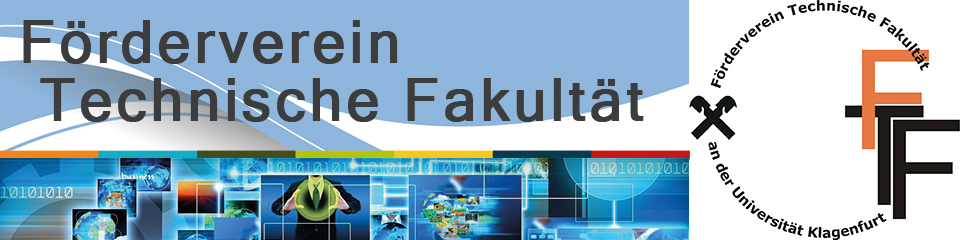## Numerical simulation for electrical tomography sensor systems and robust parameter estimation using stochastic methods

Electrical capacitance tomography (ECT) belongs to the class of so-called inverse problems which are the most challenging class of estimation problems due to their ill-posedness and their typically high-dimensional parameter spaces. In particular, ECT aims at determining electrical properties (the permittivity) of heterogeneous material distributions in inaccessible objects given sparse measurements. In order to obtain robust estimates as well as quantitative statements on parameter variability, additional information in form of prior knowledge about the unknown quantities is required. Such prior information might include system dynamics, empirical and analytical measurement models, the measurement noise mode, and certain constraints.

This talk addresses different numerical sensor modeling techniques and appropriate estimation approaches of determining process parameters in the presence of uncertainties by example. Uncertainty in recovered parameters arises from measurement noise, measurement sensitivities, model inaccuracy, discretization error, and a-priori uncertainty.

In this context, the ECT parameter estimation problem is formulated in a Bayesian inferential framework, by specifying a physically motivated prior distribution, and characterizing the statistics of measurement noise, to give a posterior distribution conditioned on measured data. The ECT sensor is simulated numerically by means of the finite element and the boundary element method. Markov chain Monte Carlo (MCMC) sampling with Metropolis-Hastings dynamics and particle filtering, notably useful for non-stationary problems, are investigated in terms of efficient exploration of the posterior distribution.

A key difference between the proposed statistical (Bayesian) approach and classical deterministic methods (e.g. Newton-type regularized optimization techniques) is that whereas regularization gives point estimates, typically using a data-misfit criterion, Bayesian methods present averages over all solutions consistent with the data. This leads to a marked difference in robustness of properties calculated from solutions. The proposed approaches are discussed for a reference problem of recovering the unknown shape and position of a constant permittivity inclusion in an otherwise uniform background. Statistics calculated in the reference problem give accurate estimates of inclusion area, and other properties, when using measured data.